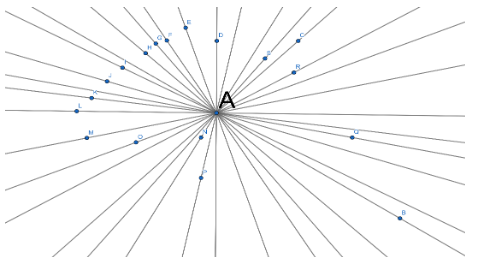Courses
Courses for Kids
Free study material
Free LIVE classes
MoreLIVE
Join Vedantu’s FREE Mastercalss

# How many lines can pass through one given point?Verified
362.7k+ views
Hint: Take a point $A$. See whether how many lines pass through the point $A$. Also, think about how the line can pass? Try it, you will get the answer.

Complete step by step solution:So we can see in the above figure that $A$is the point.
So we can see that an infinite number of lines can pass through point $A$.
So we can say that from a point an infinite number of lines can pass.

Note: Read the question carefully. Think about it. Also, your concept regarding line should be clear. You can take any of the points as we can see in the problem point $A$is taken. So, you can take any points, it is not mandatory that you take point $A$ only.
Last updated date: 26th Sep 2023
Total views: 362.7k
Views today: 7.62k